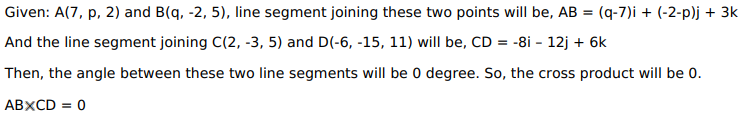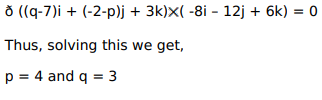# If the line segment joining the points

Question:

If the line segment joining the points $A(7, p, 2)$ and $B(q,-2,5)$ be parallel to the line segment joining the points $C(2,-3,5)$ and $D(-6,-15,11)$, find the values of $p$ and $q$.

Solution: